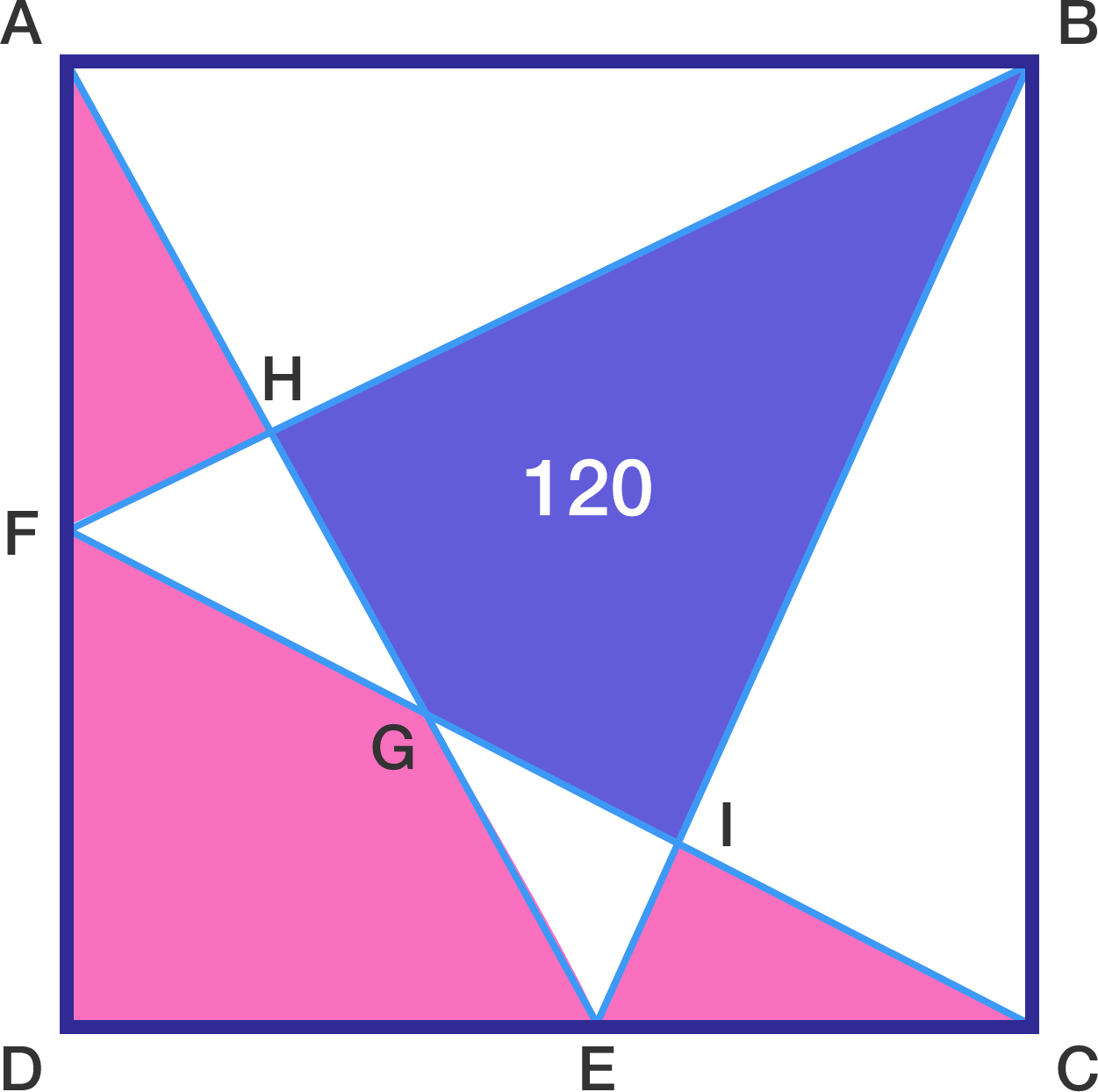# Area problem

Geometry Level 3$ABCD$ is a square with points $E$ and $F$ lying on sides $CD$ and $AD,$ respectively. If the purple area is $[BHGI]=120,$ what is the sum of the pink areas $[AHF]+[FGED]+[ICE]?$

×